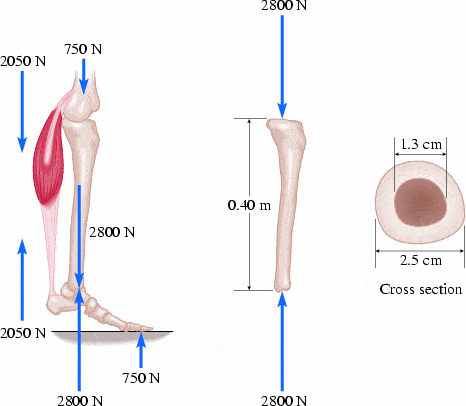# Youngs modulus stress/strain.

## Homework Statement

The figures below shows the forces acting on a tibia (shinbone, the long vertical bone in the figure) when a person stands on the ball of one foot. As shown, the force of the tibia on the ankle joint for a person (of weight 750 N) standing this way is 2800 N. The ankle joint therefore pushes upward on the bottom of the tibia with a force of 2800 N, while the top end of the tibia must feel a net downward force of approximately 2800 N (ignoring the weight of the tibia itself). The tibia has a length of 0.40 m, an average inner diameter of 1.3 cm, and an average outer diameter of 2.5 cm. (The central core of the bone contains marrow that has negligible compressive strength. Take the Young's modulus of bone to be 9.40x10^9 Pa.)(a) What is the average cross-sectional area of the tibia?

(solved 0.0003579m^2)

(b)What is the compressive stress in the tibia?

(c) What is the change in length for the tibia due to the compressive forces?

## Homework Equations

Stress is force over area

## The Attempt at a Solution

(b) should be F/0.0003579 m^2. My problem seems to be that I am having a hard time determining the forces acting on the tibia. Could anyone help?

(c) change in length is calculated by delta L = (F/A)(L/Y) where A= 0.0003579 m^2, L=.4 m and Y=9.40x10^9 Pa. Again I am uncertain of the forces acting upon the tibia so I am unable to solve.

Any suggestions?